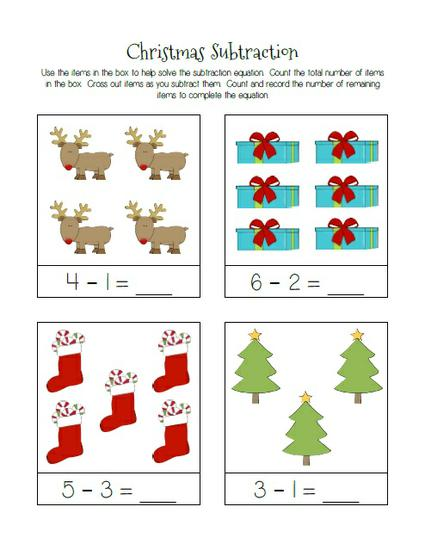# Christmas Themed Subtraction Practice Worksheets

With their colorful and cutesy Christmas themed images, these subtraction practice worksheets would make a great addition to your morning work or math centers this holiday season! Because your kiddos may be at different skill levels, along with two different types of activities to help students make important connections, each activity is also split into two worksheets - one for intermediate and one for advanced skill levels.## Picture Story Problems

The first set of worksheets invites students to look at/count the number of Christmas items in each story problem and write the appropriate subtraction equation on the lines below the pictuers.## Count & Cross Out Problems

The second set of worksheets invites students to read the subtraction equation, then use the Christmas images in the box to help them solve the equation.

• First, students count the total number of items in the box, making sure the total matches the first number in the equation.
• Next, students identify how many objects should be subtracted and physically cross out items as they subtract them.
• Last, to solve the problem, students count the remaining items in the box and fill in the equation.

## Christmas Themed Subtraction Practice Worksheets

FREE

• Delivered instantly via email
• Secure Checkout